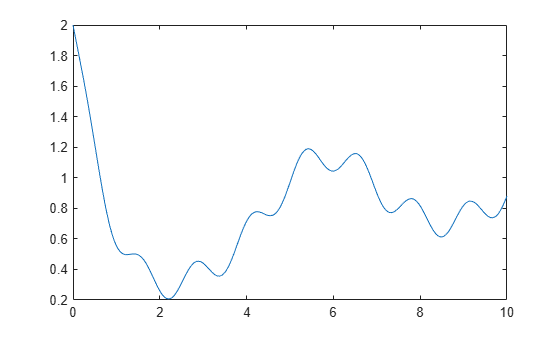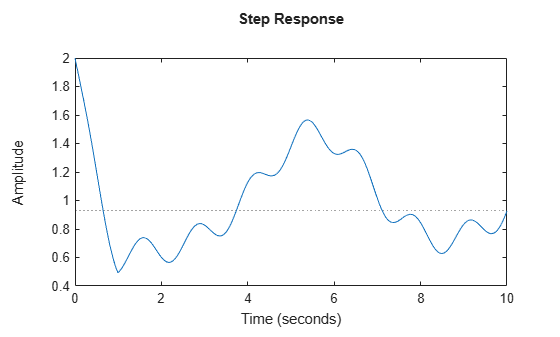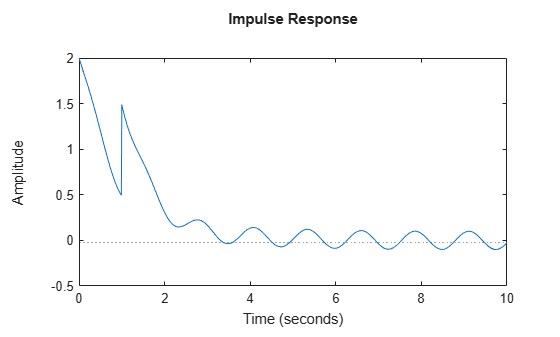# ltvss

Linear time-varying state-space model

Since R2023a

## Description

Use `ltvss` to represent linear state-space models whose dynamics vary with time.

You can use `ltvss` to create continuous-time or discrete-time linear parameter-varying state-space models. In continuous time, an `ltvss` model is described by the following state-space equations.

`$\begin{array}{c}E\left(t\right)\stackrel{˙}{x}={\delta }_{0}\left(t\right)+A\left(t\right)\left(x-{x}_{0}\left(t\right)\right)+B\left(t\right)\left(u-{u}_{0}\left(t\right)\right)\\ y\left(t\right)={y}_{0}\left(t\right)+C\left(t\right)\left(x-{x}_{0}\left(t\right)\right)+D\left(t\right)\left(u-{u}_{0}\left(t\right)\right).\end{array}$`

Here, A(t), B(t), C(t), D(t), and E(t) are time-varying state-space matrices and δ0(t), x0(t), u0(t), and y0(t) are time-dependent derivative, state, input, and output offsets, respectively.

In discrete time, an `ltvss` model is described by the following state-space equations.

`$\begin{array}{c}{E}_{k}{x}_{k+1}={\delta }_{0k}+{A}_{k}\left({x}_{k}-{x}_{0}{}_{k}\right)+{B}_{k}\left({u}_{k}-{u}_{0}{}_{k}\right)\\ {y}_{k}={y}_{0k}+{C}_{k}\left({x}_{k}-{x}_{0}{}_{k}\right)+{D}_{k}\left({u}_{k}-{u}_{0}{}_{k}\right)\end{array}$`

Here, the integer index k counts the number of sampling periods `Ts`.

You can use an `ltvss` object to model:

• Linear systems whose coefficients vary in time.

• Nonlinear systems operating along a specific trajectory. For an example, see LTV Model of Two-Link Robot.

• Nonlinear systems operating near steady-state conditions that change in time.

Use `ltvss` to construct LTV models whose dynamics are described by a MATLAB® function (the data function). Use `ssInterpolant` to construct LTV models that interpolate LTI snapshots as a function of time. See LPV and LTV Models for functions and operations applicable to `ltvss` objects.

## Creation

### Syntax

``ltvSys = ltvss(DataFcn)``
``ltvSys = ltvss(DataFcn,ts)``
``ltvSys = ltvss(DataFcn,ts,tcheck)``
``ltvSys = ltvss(___,Name=Value)``

### Description

example

````ltvSys = ltvss(DataFcn)` creates a continuous-time LTV model. `DataFcn` is the name of or a handle to the data function, the user-defined MATLAB function that calculates the matrices and offsets for given t or k values.```

example

````ltvSys = ltvss(DataFcn,ts)` creates a discrete-time LTV model with sample time `ts`.```
````ltvSys = ltvss(DataFcn,ts,tcheck)` evaluates `DataFcn` at `tcheck` to determine the number of states, inputs, and outputs. By default, `ltvss` uses `tcheck` = 0.```

example

````ltvSys = ltvss(___,Name=Value)` sets properties of the linear time-varying model using one or more name-value pair arguments. Use this syntax with any of the previous input-argument combinations.```

### Input Arguments

expand all

User-defined MATLAB function for calculating matrices and offsets, specified as a function name (character vector or string) or a function handle. The data function must be of the following form.

• Continuous time — $\left[A,B,C,D,E,{\delta }_{0},{x}_{0},{u}_{0},{y}_{0},Delays\right]=f\left(t\right)$.

• Discrete time — $\left[A,B,C,D,E,{\delta }_{0},{x}_{0},{u}_{0},{y}_{0},Delays\right]=f\left(k\right)$

For R2023a, set Delays to `[]`. In future releases, this argument will allow you to specify model delays.

This input sets the value of property `DataFunction`.

Sample time, specified as a scalar. For more information, see the `Ts` property.

Test value for time, specified as a scalar. The object evaluates `DataFcn` at `tcheck` to check if it is valid and to obtain the number of states, inputs, and outputs. By default, `ltvss` uses `tcheck` = 0.

## Properties

expand all

Data function for calculating the model data, specified as a function handle. The data function must be of the following form.

• Continuous time

`[A,B,C,D,E,dx0,x0,u0,y0,Delay] = DataFcn(t)`
• Discrete time

`[A,B,C,D,E,dx0,x0,u0,y0,Delay] = DataFcn(k)`

Here, the input `k` is an integer index that counts the number of sampling periods `Ts`. The absolute time is given by `t` = `k*Ts`.

To pass additional input arguments, use an anonymous function as follows.

`DataFcn = @(t) myFunction(t,arg1,arg2,...)`

You can set all output arguments except `A`, `B`, `C`, `D` to `[]` when absent for `t` values.

State names, specified as one of the following:

• Character vector — For first-order models, for example, `'velocity'`.

• Cell array of character vectors — For models with two or more states

`StateName` is empty `' '` for all states by default.

State path to facilitate state block path management in linearization, specified as one of the following:

• Character vector — For first-order models

• Cell array of character vectors — For models with two or more states

`StatePath` is empty `' '` for all states by default.

State units, specified as one of the following:

• Character vector — For first-order models, for example, `'m/s'`

• Cell array of character vectors — For models with two or more states

Use `StateUnit` to keep track of the units of each state. `StateUnit` has no effect on system behavior. `StateUnit` is empty `' '` for all states by default.

Sample time, specified as:

• `0` for continuous-time systems.

• A positive scalar representing the sampling period of a discrete-time system. Specify `Ts` in the time unit specified by the `TimeUnit` property.

• `-1` for a discrete-time system with an unspecified sample time.

Note

Changing `Ts` does not discretize or resample the model. To convert between continuous-time and discrete-time representations, use `c2d` and `d2c`. To change the sample time of a discrete-time system, use `d2d`.

Time variable units, specified as one of the following:

• `'nanoseconds'`

• `'microseconds'`

• `'milliseconds'`

• `'seconds'`

• `'minutes'`

• `'hours'`

• `'days'`

• `'weeks'`

• `'months'`

• `'years'`

Changing `TimeUnit` has no effect on other properties, but changes the overall system behavior. Use `chgTimeUnit` to convert between time units without modifying system behavior.

Input channel names, specified as one of the following:

• A character vector, for single-input models.

• A cell array of character vectors, for multi-input models.

• `''`, no names specified, for any input channels.

Alternatively, you can assign input names for multi-input models using automatic vector expansion. For example, if `sys` is a two-input model, enter the following:

`sys.InputName = 'controls';`

The input names automatically expand to `{'controls(1)';'controls(2)'}`.

You can use the shorthand notation `u` to refer to the `InputName` property. For example, `sys.u` is equivalent to `sys.InputName`.

Use `InputName` to:

• Identify channels on model display and plots.

• Extract subsystems of MIMO systems.

• Specify connection points when interconnecting models.

Input channel units, specified as one of the following:

• A character vector, for single-input models.

• A cell array of character vectors, for multi-input models.

• `''`, no units specified, for any input channels.

Use `InputUnit` to specify input signal units. `InputUnit` has no effect on system behavior.

Input channel groups, specified as a structure. Use `InputGroup` to assign the input channels of MIMO systems into groups and refer to each group by name. The field names of `InputGroup` are the group names and the field values are the input channels of each group. For example, enter the following to create input groups named `controls` and `noise` that include input channels `1` and `2`, and `3` and `5`, respectively.

```sys.InputGroup.controls = [1 2]; sys.InputGroup.noise = [3 5];```

You can then extract the subsystem from the `controls` inputs to all outputs using the following.

`sys(:,'controls')`

By default, `InputGroup` is a structure with no fields.

Output channel names, specified as one of the following:

• A character vector, for single-output models.

• A cell array of character vectors, for multi-output models.

• `''`, no names specified, for any output channels.

Alternatively, you can assign output names for multi-output models using automatic vector expansion. For example, if `sys` is a two-output model, enter the following.

`sys.OutputName = 'measurements';`

The output names automatically expand to `{'measurements(1)';'measurements(2)'}`.

You can also use the shorthand notation `y` to refer to the `OutputName` property. For example, `sys.y` is equivalent to `sys.OutputName`.

Use `OutputName` to:

• Identify channels on model display and plots.

• Extract subsystems of MIMO systems.

• Specify connection points when interconnecting models.

Output channel units, specified as one of the following:

• A character vector, for single-output models.

• A cell array of character vectors, for multi-output models.

• `''`, no units specified, for any output channels.

Use `OutputUnit` to specify output signal units. `OutputUnit` has no effect on system behavior.

Output channel groups, specified as a structure. Use `OutputGroup`to assign the output channels of MIMO systems into groups and refer to each group by name. The field names of `OutputGroup` are the group names and the field values are the output channels of each group. For example, create output groups named `temperature` and `measurement` that include output channels `1`, and `3` and `5`, respectively.

```sys.OutputGroup.temperature = ; sys.OutputGroup.measurement = [3 5];```

You can then extract the subsystem from all inputs to the `measurement` outputs using the following.

`sys('measurement',:)`

By default, `OutputGroup` is a structure with no fields.

System name, specified as a character vector. For example, `'system_1'`.

User-specified text that you want to associate with the system, specified as a character vector or cell array of character vectors. For example, `'System is MIMO'`.

User-specified data that you want to associate with the system, specified as any MATLAB data type.

## Object Functions

expand all

 `sample` Sample linear parameter-varying or time-varying dynamics `ssInterpolant` Build gridded LTV or LPV model from state-space data
 `step` Step response plot of dynamic system; step response data `impulse` Impulse response plot of dynamic system; impulse response data `lsim` Plot simulated time response of dynamic system to arbitrary inputs; simulated response data `initial` System response to initial states of state-space model
 `feedback` Feedback connection of multiple models `connect` Block diagram interconnections of dynamic systems `series` Series connection of two models `parallel` Parallel connection of two models `lft` Generalized feedback interconnection of two models (Redheffer star product)
 `c2d` Convert model from continuous to discrete time `d2c` Convert model from discrete to continuous time `d2d` Resample discrete-time model `xperm` Reorder states in state-space models `sminreal` Structural pole/zero cancellations

## Examples

collapse all

Create a continuous-time SISO linear-time varying model.

This example uses a first-order model. The matrices and offsets are given by:

$\mathit{A}=-\left(1+0.5\mathrm{sin}\left(\mathit{t}\right)\right)$, $\mathit{B}=1$, $\mathit{C}=1$, $\mathit{D}=0$,

${\mathit{y}}_{0}=0.1\mathrm{sin}\left(5\mathit{t}\right)$.

These matrices and offsets are defined in the `ltvssDataFcn.m` data function provided with this example.

Create the LTV model.

`ltvSys = ltvss(@ltvssDataFcn)`
```Continuous-time state-space LTV model with 1 outputs, 1 inputs, and 1 states. ```

View the data function.

`type ltvssDataFcn.m`
```function [A,B,C,D,E,dx0,x0,u0,y0,Delays] = ltvssDataFcn(t) % SISO, first order A = -(1+0.5*sin(t)); B = 1; C = 1; D = 0; E = []; dx0 = []; x0 = []; u0 = []; y0 = 0.1*sin(5*t); Delays = []; ```

Create a discrete-time linear-parameter varying model.

This example uses a model with the matrices and offsets defined as:

$\mathit{A}=\mathrm{sin}\left(0.1\mathit{k}\right)$, $\mathit{B}=1$, $\mathit{C}=1$, $\mathit{D}=0$

`${\mathit{y}}_{0}=0.1\mathrm{sin}\left(\mathit{k}\right)$`

These matrices and offsets are defined in the `ltvFcnDiscrete.m` data function provided with this example.

Specify the properties and create the LTV model.

```Ts = 0.01; DataFcn = @ltvFcnDiscrete; ltvSys = ltvss(DataFcn,Ts)```
```Discrete-time state-space LTV model with 1 outputs, 1 inputs, and 1 states. ```

You can set additional properties of the model using the dot notation

```ltvSys.InputName = 'u'; ltvSys.OutputName = 'y';```

View the data function.

`type ltvFcnDiscrete.m`
```function [A,B,C,D,E,dx0,x0,u0,y0,Delays] = ltvFcnDiscrete(k) A = sin(0.1*k); B = 1; C = 1; D = 0; E = []; dx0 = []; x0 = []; u0 = []; y0 = 0.1*sin(k); Delays = []; ```

For this example, consider `ltvssDataFcn.m` that defines the matrices and offsets of a MIMO system.

Create The LTV model.

`ltvSys = ltvss(@ltvssDataFcn);`

Simulate the response of this model from t = 0 to t = 10 seconds to an arbitrary input.

```t = (0:.01:10)'; u = sin(0.2*t); x0 = 2;```

Simulate and plot the response.

```[y,~,x] = lsim(ltvSys,u,t,x0); plot(t,y)```Now, simulate the model to step and impulse responses.

Create the option set using `RespConfig` to specify initial offset, and state values.

`respOpt = RespConfig(InitialState=x0,Delay=1);`

Compute the step response.

`step(ltvSys,t,respOpt)`Compute the impulse response.

`impulse(ltvSys,t,respOpt)`View the data function.

`type ltvssDataFcn.m`
```function [A,B,C,D,E,dx0,x0,u0,y0,Delays] = ltvssDataFcn(t) % SISO, first order A = -(1+0.5*sin(t)); B = 1; C = 1; D = 0; E = []; dx0 = []; x0 = []; u0 = []; y0 = 0.1*sin(5*t); Delays = []; ```

## Version History

Introduced in R2023a